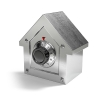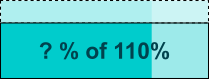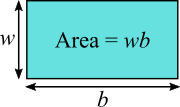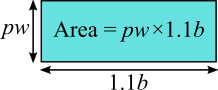You may also likeCD Heaven

All CD Heaven stores were given the same number of a popular CD to sell for £24. In their two week sale each store reduces the price of the CD by 25% ... How many CDs did the store sell at each price?A Change in Code

There are two sets of numbers. The second is the result of the first after an increase by a constant percentage. How can you find that percentage if one set of numbers is in code?Pied Piper

How many rats did the Pied Piper catch?

Percentage Unchanged

Age 14 to 16 Short Challenge Level:

Using scale factorsArea is unchanged so ?% of 110% = 100%
\begin{align} \tfrac{?}{100}\times110 &= 100\\ ?\times110&=10000\\ ?\times11&=1000\\ ? &= \tfrac{1000}{11} \text{ or } 90.90909...\end{align} So the width is decreased by $100-90.90909...=9.090909...\text{ or }\frac{100}{11}=9\frac1{11}$

Using algebra
The area of the original rectangle was $wb$The area of the altered rectangle is $pw \times 1.1b$Since the two areas are equal,

$1.1 pwb = wb$

so

$1.1 p = 1$

so $p = \frac{1}{1.1} = \frac{10}{11}$

So the width is $\frac{10}{11}$ of its original value, so it has been decreased by $\frac1{11}$, which as a percentage is $9$ and $\frac1{11}\%$, or $9.09\%$ to 2.d.p.

You can find more short problems, arranged by curriculum topic, in our short problems collection.